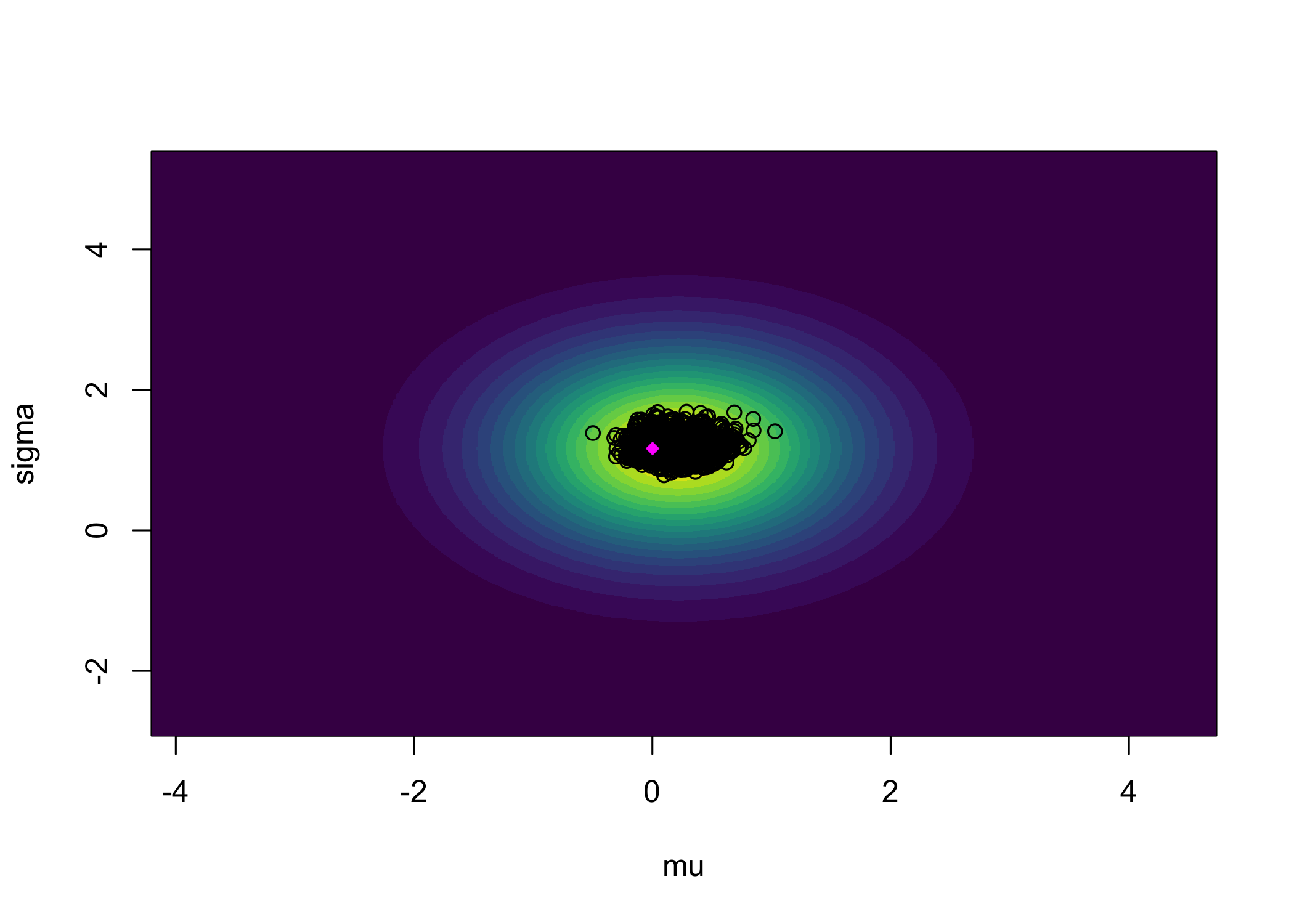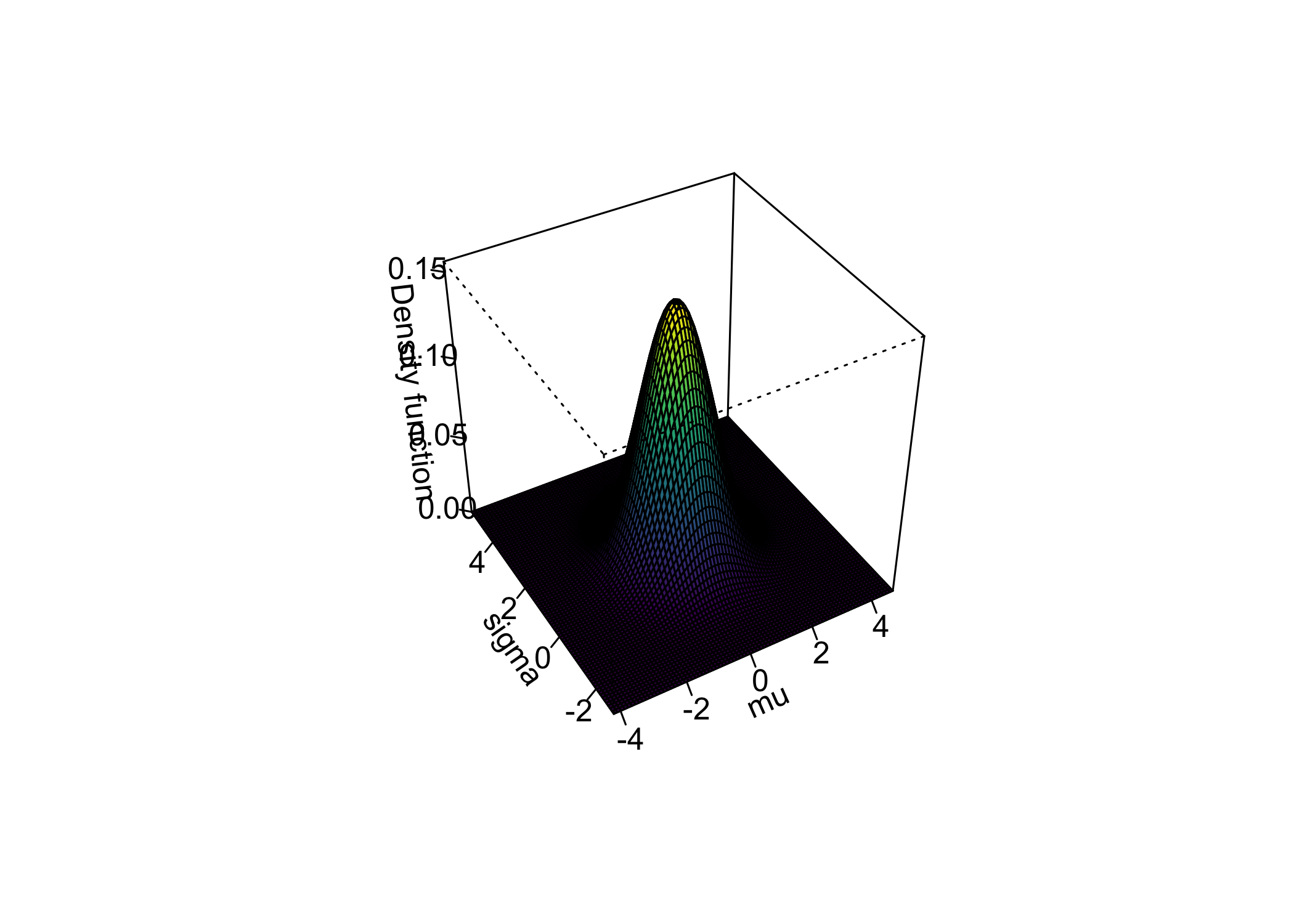# Precise Bayesian hypothesis testing with the Full Bayesian Significance Test in multidimensional posteriors

## Precise Bayesian hypothesis testing with the Full Bayesian Significance Test in multidimensional posteriors

Author: Riko Kelter

This vignette explains how to use the Full Bayesian Significance Test (FBST) for Bayesian hypothesis testing of a precise (point-null) hypothesis against its alternative via the e-value in posteriors of dimension larger than one.

library(fbst)

The theory behind the FBST and e-value has been detailed in the vignette ‘Precise Bayesian hypothesis testing with the Full Bayesian Significance Test’. In this vignette, we illustrate how the FBST can be applied when the dimension of the posterior is of dimension larger than one. In a broad variety of models, the posterior has multiple parameters of interest, for example, take a simple normal model $Y \sim N(\mu,\sigma^2)$ where we use a normal prior for the mean $$\mu$$ and an exponential prior for the standard deviation $$\sigma$$: $\mu \sim N(0,1), \hspace{0.5cm} \sigma \sim \exp(1)$ Now, the full-dimensional posterior when both $$\mu$$ and $$\sigma$$ are unknown is given as $p(\mu,\sigma|y)$ given the observed data $$y$$. Thus, in such cases it is not allowed to shift to a marginal posterior $p(\mu|y)=\int p(\mu,\sigma|y)p(\sigma)d\sigma$ of the parameter $$\mu$$ of interest and calculate the supremum of the surprise function under the null set $$\Theta_{H_0}$$ based on this marginal posterior $$p(\mu|y)$$. Instead, one must use the two-dimensional posterior $$p(\mu,\sigma|y)$$ in such cases.

Therefore, we use a linear regression model without a predictor, so we are left with the standard deviation $$\sigma$$ and the intercept, which is the mean parameter $$\mu$$. Now, we fit the model:

library(rstanarm)
set.seed(42)
mu_true = 0.25
sigma_true = 1
stanData = data.frame(y=rnorm(50,mu_true,sigma_true))
fit1 <- stan_glm(y ~ NULL, data = stanData,
prior_intercept = normal(0,10),
prior_aux = exponential(1),
seed = 12345)
#>
#> SAMPLING FOR MODEL 'continuous' NOW (CHAIN 1).
#> Chain 1:
#> Chain 1: Gradient evaluation took 5.7e-05 seconds
#> Chain 1: 1000 transitions using 10 leapfrog steps per transition would take 0.57 seconds.
#> Chain 1:
#> Chain 1:
#> Chain 1: Iteration:    1 / 2000 [  0%]  (Warmup)
#> Chain 1: Iteration:  200 / 2000 [ 10%]  (Warmup)
#> Chain 1: Iteration:  400 / 2000 [ 20%]  (Warmup)
#> Chain 1: Iteration:  600 / 2000 [ 30%]  (Warmup)
#> Chain 1: Iteration:  800 / 2000 [ 40%]  (Warmup)
#> Chain 1: Iteration: 1000 / 2000 [ 50%]  (Warmup)
#> Chain 1: Iteration: 1001 / 2000 [ 50%]  (Sampling)
#> Chain 1: Iteration: 1200 / 2000 [ 60%]  (Sampling)
#> Chain 1: Iteration: 1400 / 2000 [ 70%]  (Sampling)
#> Chain 1: Iteration: 1600 / 2000 [ 80%]  (Sampling)
#> Chain 1: Iteration: 1800 / 2000 [ 90%]  (Sampling)
#> Chain 1: Iteration: 2000 / 2000 [100%]  (Sampling)
#> Chain 1:
#> Chain 1:  Elapsed Time: 0.013896 seconds (Warm-up)
#> Chain 1:                0.017853 seconds (Sampling)
#> Chain 1:                0.031749 seconds (Total)
#> Chain 1:
#>
#> SAMPLING FOR MODEL 'continuous' NOW (CHAIN 2).
#> Chain 2:
#> Chain 2: Gradient evaluation took 6e-06 seconds
#> Chain 2: 1000 transitions using 10 leapfrog steps per transition would take 0.06 seconds.
#> Chain 2:
#> Chain 2:
#> Chain 2: Iteration:    1 / 2000 [  0%]  (Warmup)
#> Chain 2: Iteration:  200 / 2000 [ 10%]  (Warmup)
#> Chain 2: Iteration:  400 / 2000 [ 20%]  (Warmup)
#> Chain 2: Iteration:  600 / 2000 [ 30%]  (Warmup)
#> Chain 2: Iteration:  800 / 2000 [ 40%]  (Warmup)
#> Chain 2: Iteration: 1000 / 2000 [ 50%]  (Warmup)
#> Chain 2: Iteration: 1001 / 2000 [ 50%]  (Sampling)
#> Chain 2: Iteration: 1200 / 2000 [ 60%]  (Sampling)
#> Chain 2: Iteration: 1400 / 2000 [ 70%]  (Sampling)
#> Chain 2: Iteration: 1600 / 2000 [ 80%]  (Sampling)
#> Chain 2: Iteration: 1800 / 2000 [ 90%]  (Sampling)
#> Chain 2: Iteration: 2000 / 2000 [100%]  (Sampling)
#> Chain 2:
#> Chain 2:  Elapsed Time: 0.01541 seconds (Warm-up)
#> Chain 2:                0.016326 seconds (Sampling)
#> Chain 2:                0.031736 seconds (Total)
#> Chain 2:
#>
#> SAMPLING FOR MODEL 'continuous' NOW (CHAIN 3).
#> Chain 3:
#> Chain 3: Gradient evaluation took 6e-06 seconds
#> Chain 3: 1000 transitions using 10 leapfrog steps per transition would take 0.06 seconds.
#> Chain 3:
#> Chain 3:
#> Chain 3: Iteration:    1 / 2000 [  0%]  (Warmup)
#> Chain 3: Iteration:  200 / 2000 [ 10%]  (Warmup)
#> Chain 3: Iteration:  400 / 2000 [ 20%]  (Warmup)
#> Chain 3: Iteration:  600 / 2000 [ 30%]  (Warmup)
#> Chain 3: Iteration:  800 / 2000 [ 40%]  (Warmup)
#> Chain 3: Iteration: 1000 / 2000 [ 50%]  (Warmup)
#> Chain 3: Iteration: 1001 / 2000 [ 50%]  (Sampling)
#> Chain 3: Iteration: 1200 / 2000 [ 60%]  (Sampling)
#> Chain 3: Iteration: 1400 / 2000 [ 70%]  (Sampling)
#> Chain 3: Iteration: 1600 / 2000 [ 80%]  (Sampling)
#> Chain 3: Iteration: 1800 / 2000 [ 90%]  (Sampling)
#> Chain 3: Iteration: 2000 / 2000 [100%]  (Sampling)
#> Chain 3:
#> Chain 3:  Elapsed Time: 0.013952 seconds (Warm-up)
#> Chain 3:                0.018139 seconds (Sampling)
#> Chain 3:                0.032091 seconds (Total)
#> Chain 3:
#>
#> SAMPLING FOR MODEL 'continuous' NOW (CHAIN 4).
#> Chain 4:
#> Chain 4: Gradient evaluation took 6e-06 seconds
#> Chain 4: 1000 transitions using 10 leapfrog steps per transition would take 0.06 seconds.
#> Chain 4:
#> Chain 4:
#> Chain 4: Iteration:    1 / 2000 [  0%]  (Warmup)
#> Chain 4: Iteration:  200 / 2000 [ 10%]  (Warmup)
#> Chain 4: Iteration:  400 / 2000 [ 20%]  (Warmup)
#> Chain 4: Iteration:  600 / 2000 [ 30%]  (Warmup)
#> Chain 4: Iteration:  800 / 2000 [ 40%]  (Warmup)
#> Chain 4: Iteration: 1000 / 2000 [ 50%]  (Warmup)
#> Chain 4: Iteration: 1001 / 2000 [ 50%]  (Sampling)
#> Chain 4: Iteration: 1200 / 2000 [ 60%]  (Sampling)
#> Chain 4: Iteration: 1400 / 2000 [ 70%]  (Sampling)
#> Chain 4: Iteration: 1600 / 2000 [ 80%]  (Sampling)
#> Chain 4: Iteration: 1800 / 2000 [ 90%]  (Sampling)
#> Chain 4: Iteration: 2000 / 2000 [100%]  (Sampling)
#> Chain 4:
#> Chain 4:  Elapsed Time: 0.01392 seconds (Warm-up)
#> Chain 4:                0.018353 seconds (Sampling)
#> Chain 4:                0.032273 seconds (Total)
#> Chain 4:

We can print the fit:

print(fit1)
#> stan_glm
#>  family:       gaussian [identity]
#>  formula:      y ~ NULL
#>  observations: 50
#>  predictors:   1
#> ------
#> (Intercept) 0.2    0.2
#>
#> Auxiliary parameter(s):
#> sigma 1.2    0.1
#>
#> ------
#> * For help interpreting the printed output see ?print.stanreg
#> * For info on the priors used see ?prior_summary.stanreg

We store the posterior draws of $$\mu$$ and $$\sigma$$ in a matrix:

posteriorDrawsMatrix = as.matrix(fit1)
#>           parameters
#> iterations (Intercept)     sigma
#>       [1,]  0.18938525 1.1507643
#>       [2,]  0.18818783 1.1706089
#>       [3,]  0.07120997 1.1807322
#>       [4,]  0.33424904 1.1841644
#>       [5,]  0.25017576 1.1869898
#>       [6,]  0.30096634 0.9417595

Now we call the fbst function:

resFlat = fbst(posteriorDrawsMatrix, nullHypothesisValue=0, dimensionTheta=2, dimensionNullset=1, dim = 2, gridSize = 1000)
summary(resFlat)
#> Full Bayesian Significance Test for testing a sharp hypothesis against its alternative:
#> Reference function: Flat
#> Hypothesis H_0:Parameter= 0 against its alternative H_1
#> Bayesian e-value against H_0: 0.682
#> Standardized e-value: 0.1300919

The plain e-value against the null hypothesis $$H_0:\mu=0$$ can be accessed as follows:

resFlat$eValue #> eValue #> 0.682 In the two-dimensional case, visualization of the results is possible via contour plots or perspective plots: plot(resFlat, type = "contour", parNames=c("mu","sigma"))The above contour plot shows the posterior draws as black points, and the parameter value $$(mu_0,\hat{\sigma})$$ (magenta point) which maximizes the surprise function under the null set $$\Theta_{H_0}:=\{(0,\sigma)|\sigma \in \mathbb{R}_{+}\}$$. The e-value against $$H_0:\mu=0$$ is the integral over the two-dimensional posterior for which the posterior surprise function values $\frac{p(\mu,\sigma|y)}{r(\mu,\sigma)}\geq \frac{p(\mu_0,\hat{\sigma}|y)}{r(\mu,\sigma)}$ Here, we have used a flat reference function $$r(\mu,\sigma):=1$$, and this is currently the only option in the fbst package when two-dimensional posteriors are used. Note further that as base R does not support multidimensional Gaussian kernel density estimation, the fbst package relies on the ks package for this task, and supports only posteriors up to dimension two. plot(resFlat, type="persp", parNames=c("mu","sigma")) #> Warning in system2("/usr/bin/otool", c("-L", shQuote(DSO)), stdout = TRUE): #> Ausführung von Kommando ''/usr/bin/otool' -L '/Library/Frameworks/R.framework/ #> Resources/library/tcltk/libs//tcltk.so'' ergab Status 1The above plot shows the posterior surface for different values of $$(\mu,\sigma)$$ based on the simulated data. The evidence against the precise hypothesis $$H_0:\mu=0$$ is given as resFlat$eValue
#> eValue
#>  0.682

The evidence against $$H_0:\mu=0$$ is thus quite large. Note that the standardized e-value can be obtained as in the one-dimensional posterior case:

resFlat\$sev_H_0
#>   sev_H_0
#> 0.1300919

Thus, based on the standardized e-value which makes use of asymptotic arguments we would reject the null hypothesis $$H_0:\mu=0$$.